### Equations used in the NMR tutorial

(see below for some sample calculations)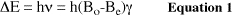where:

•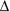E is the energy difference between spin states
• h is Planck's constant
•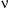is the frequency of the B1 field
• Be is a small magnetic field generated by the circulation of electrons of the molecule (see below)
• Bo is the strength of the external homogeneous magnetic field
•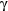(gyromagnetic ratio) is a constant which is a property of the particular nucleus

(Discussed in Spin states)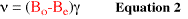(obtained by dividing eq 1 by h)

(Discussed in Spin states)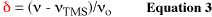• (-TMS) is the frequency difference between the resonance of the signal of interest and the resonance of TMS. Note that (-TMS) will always be in units of Hz (from 1 Hz to a few thousand Hz).
•is the center frequency of the B1 field in megahertz (MHz).
• If Bo = 7.05 T,= 300 MHz for 1H nuclei or 75 Mz for 13C nuclei.
• If Bo = 11.75 T,= 500 MHz for 1H nuclei or 125 Mz for 13C nuclei.

(Discussed in Chemical shift)

### Sample Calculations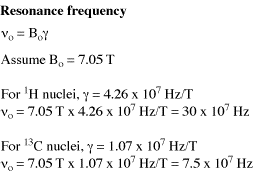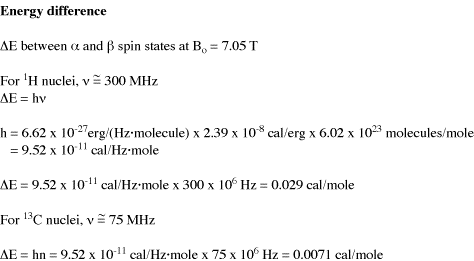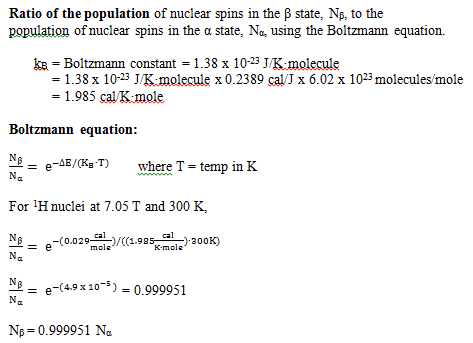back to contentsCopyright information: Original content © University of Colorado, Boulder, Chemistry and Biochemistry Department, 2011. The information on these pages is available for academic use without restriction.